Over a million developers have joined DZone.{{announcement.body}}
{{announcement.title}}

Improved R Implementation of Collaborative Filtering

DZone 's Guide to

Improved R Implementation of Collaborative Filtering

Collaborative filtering (CF) is a popular techniques for building recommender systems. It's applicable to large-scale datasets and reduces training time greatly.

· Big Data Zone ·
Free Resource

Comment (0)

Save
{{ articles.views | formatCount}} Views

Collaborative filtering (CF) is one of the most popular techniques for building recommender systems. It is a method of making automatic predictions about the interests of a user by collecting preferences or taste information from many users (collaborating). There are two main types of CF algorithms: memory-based and model-based. Very often, prediction accuracy can be improved by combining them into a single model.

In this post, we will focus on memory-based algorithms and go into details of their derivation and implementation. We will showcase some of our recent work on improving the classic CF implementation, thus making it applicable to large-scale data sets and reducing the training time by several magnitudes. Our algorithm is written in R, but it can be implemented in other languages as well.

Memory-based algorithms can be divided into:

• User-based CF: If we want to predict how user U will rate item I, we can check how other users who are similar to user U have rated that item. It is probable that the user will rate items similarly to users with similar taste.
• Item-based CF: If we want to predict how user U will rate item I, we can check how he has rated other items, which are similar to item I. It is probable that the user will rate similar items similarly.

Let's go through an example and see how user-based CF can be implemented. The following formulas show how to calculate rating ru,i, the prediction about how user u will rate item i. We aggregate over ratings that users similar to u have given to item i (the set of similar users is marked with S; the similarity function is marked with sim). The more similar a user is, the more influence his rating has to the overall prediction. The value of w is the weighting factor used to scale the sum down to a single rating.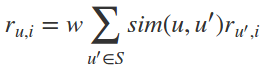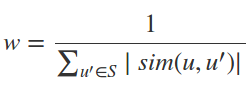In order to evaluate a recommender system, we need to calculate the predictions for all ratings in a test set. Calculations are usually not done in a loop, but rather using matrix multiplication since it is a much faster operation. The following picture shows the matrices being used: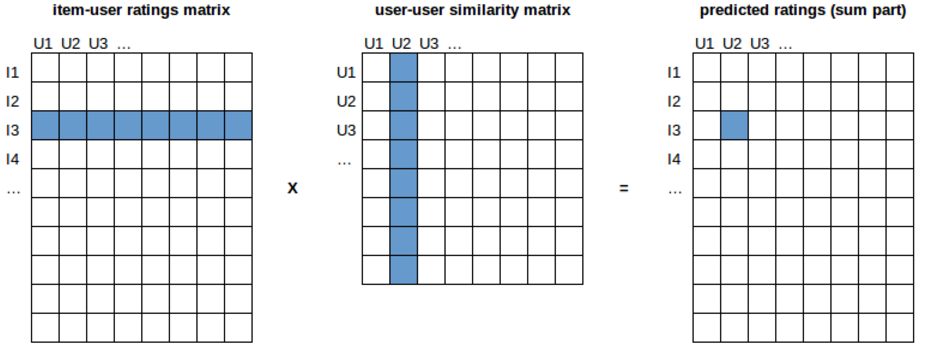Let’s focus on user U2 and item I3 for a moment. For predicting how user U2 will rate item I3, we need to know how other users have rated I3 (the blue row in the first matrix) and how much other users are similar to U2 (the blue column in the second matrix; note that the similarity of U2 to itself can be ignored by setting it to zero). In this case, the formula for the sum from above can be written as follows (user and item are marked as u2 and i3, and set S covers all users):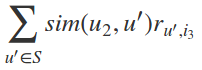The result is stored in the blue cell of the rightmost matrix. In order to find the final prediction, we also need the coefficient w (as explained above), which is calculated in a similar manner. Finally, by multiplying two matrices, we get instant results for all predictions (not only for U2, I3).

One of the main disadvantages of memory-based CF is related to its scalability and performance. For example, if there are 500,000 users and we need to implement user-based CF, it is necessary to find similarities between all pairs of users (over 120 billion values in the worst case). Obviously, this requires a lot of memory and processing time. We will try to address these issues using new implementation of CF in R programming language (this can be applied to other languages, as well).

Our implementation will be compared to one of the most commonly used packages for recommender systems in R, recommenderlab. The comparison was performed on a single computer with 4-core i7 and 16Gb RAM, using three well-known and freely available data sets (MovieLens 100k, MovieLens 1m, MovieLens 10m). It will be shown that our implementation:

1. Is significantly faster.
2. Can support building recommender systems on large data sets, for which recommenderlab implementation runs out of memory.

Execution Time Improvement

Ratings matrices are usually both large (there are a lot of users and items) and sparse (users typically rate only a few items, if any). In R, there is a special representation for sparse matrices, such that missing values (ratings) are not stored into memory. Very often, over 90% of ratings are missing, so this saves a lot of memory. Our implementation, as well as recommenderlab, uses this sparse form of matrices.

The main steps used in our implementation of user-based CF are as follows (the same approach is used for item-based CF):

1. Take a ratings matrix.
2. The user specifies if he or she wants to normalize ratings. This step usually increases accuracy. Normalization is used to remove individual ratings bias, introduced by users who consistently give lower or higher ratings compared to other users.
3. Calculate similarities between users.
4. Use the k nearest neighbor approach (keep only k most similar users, by keeping only k highest values per columns in the user-user similarity matrix). The user needs to specify the value of k.
5. Calculate predictions and denormalize them in case normalization was performed in Step 2.

The implementation in recommenderlab follows fairly the same procedure. However, we have introduced optimizations which have resulted in significant speed improvements. Two main optimization steps are summarized below:

1. The calculation of similarities is optimized for large sparse matrices. The approach is thoroughly described in our previous blog post.
2. k nearest neighbors on similarity matrices were not calculated in a loop, but rather using an optimized implementation. First, we grouped all the values from the similarity matrix by column. In each group (column), we applied a function that finds the k-th highest value. This was implemented using the R ‘data.table’ package. Finally, we used this information to keep only the k highest values per column in the similarity matrix.

Evaluation

We compared our implementation vs. recommenderlab using the following setup:

• 10-fold cross validation. In each iteration, 90% of the ratings were used to create the model and calculate similarities and 10% were used for testing. All users and items were considered for both training and testing.
• Center normalization, where user’s average rating is subtracted from his actual ratings.
• Cosine measure to calculate similarities.
• k: The number of nearest neighbors was set to 100, 300 and ALL.

The evaluation was performed on two popular data sets (MovieLens 100k and MovieLens 1m). The results can be found in the following tables, showing:

• rmse: Error that the algorithm makes (root-mean-square error).
• exec time: Execution time of the algorithm, in seconds.

Comparison on 100k MovieLens Data Set

This data set contains 943 users and 1,682 movies (items), with 100,000 ratings.

User-Based CF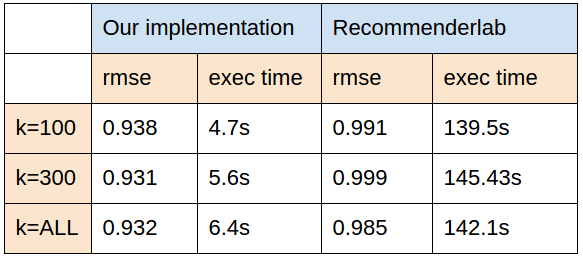Item-Based CF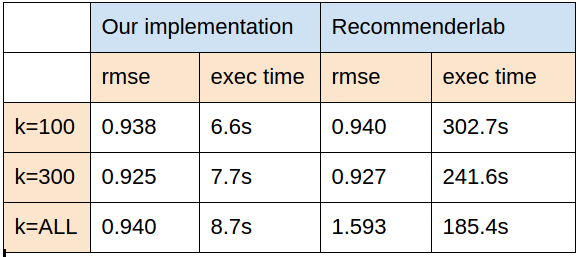Comparison on 1m MovieLens Data Set

This data set contains 6,040 users and 3,706 movies (items), with 1,000 209 ratings.

User-Based CF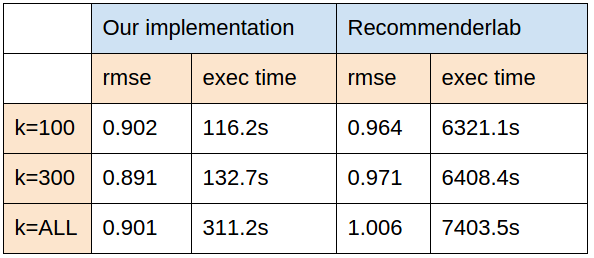Item-Based CF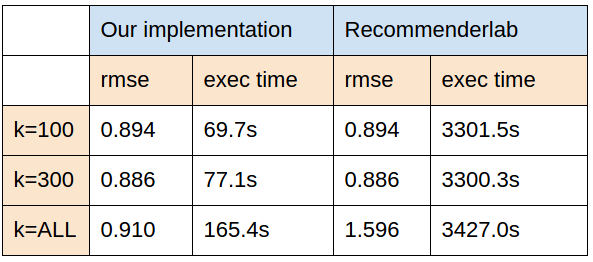It can be observed that our implementation runs much faster, and is, in addition, more accurate (achieves lower rmse). The main improvement we have achieved is a significant speed-up, the result which we wanted to achieve in the first place.

However, the speed is only one side of the problem with classic implementation. As we have already mentioned in the first section, another concern regarding CF is space, i.e. what to do when we run out of memory in case matrices become too large. In the next section, we will introduce a new approach to make it feasible to train CF recommender even on large data sets, on which classic implementation, such as those in ‘recommenderlab’, runs out of memory.

Build a Recommender on Large Data Sets

In this test, we used MovieLens 10m data set. As we have already mentioned, all algorithms were run on a single machine with 16 Gb RAM and evaluated using 10-fold cross validation. In such a setup, ‘recommenderlab’ implementation can not be used on this data set (at least for user-based CF, since it runs out of memory when similarities matrix needs to be calculated).

In our implementation, we tried to solve the problem of large matrices by dividing them into parts, i.e., we did not calculate all predictions at once, but in chunks. Here is the procedure for user-based CF (the same approach is used for item-based CF):

1. Take N rows (items) of item-user matrix. In the picture, we took rows of indices [I1:I4]
2. Take M users and calculate similarities between them and all other users. In the picture, we calculated similarities for users [U1:U2].
3. Calculate the product of N rows from Step 1 and M columns from Step 2. The result is MXN matrix that contains the sums used to find predictions. In our example, those will be predictions about how items I1 to I4 will be rated by users U1 and U2.
4. Repeat the first three steps for different N and M chunks until the result matrix is fully covered.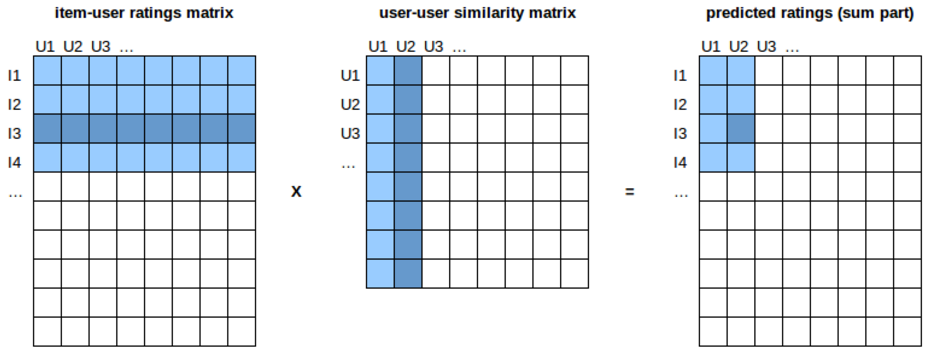Results on MovieLens 10m Data Set

This data set contains 69,878 users and 10,677 movies, with around 10,000,054 ratings. We have tested our algorithm using the following evaluation setup:

• 10-fold cross validation, as explained above.
• Center normalization.
• Cosine measure to calculate similarities.
• k: The number of nearest neighbors was set to 100 and 1,000.
• Chunk size: The numbers of rows and columns per chunk are the parameters of the algorithm. We used some values we found to be nearly optimal for our hardware setup.

The results of the comparison can be found in following tables, showing:

• rmse: Error that algorithm makes (root-mean-square error)
• exec time: Execution time of the algorithm, in minutes.
• num predictions: Number of predictions CF was able to calculate. In general, CF cannot always calculate a prediction (i.e., if a user has no similar users who have rated the given item).

Item-Based CF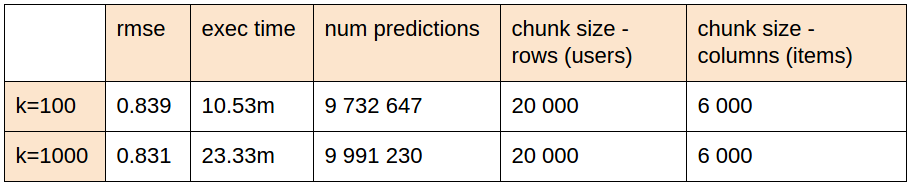User-Based CF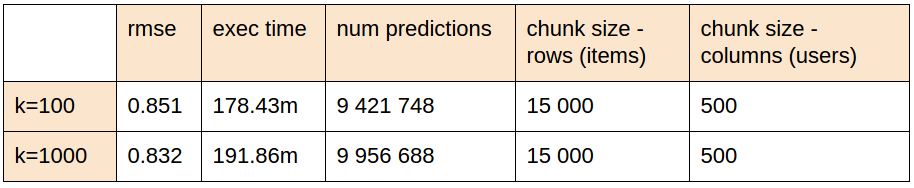As we can observe, the algorithm was executed successfully. Now it is feasible to build a CF recommender for this data set even on a modest machine configuration with 16Gb RAM. The evaluation typically takes between 10min and 200min to finish a 10-fold cross validation. User-based CF requires more time because there are more users and more similarities to calculate.

With this current implementation, when we need to find recommendations in real-time for one or several users, the calculation of similarities and predictions will be much faster since we will operate on a small number of users. On MovieLens 10m data set, user-based CF takes a second to find predictions for one or several users, while item-based CF takes around 30 seconds because of the time needed to calculate the similarity matrix. This can be optimized further, by storing the similarity matrix as a model, rather than calculating it on-fly. An obvious advantage of this algorithm is that it is scalable. Since we calculate predictions on chunks of matrices independently, it is suitable to be parallelized. One of our next steps is to implement and test this approach on some distributed framework.

Conclusion

In this blog, we presented a novel approach to improve existing implementations of memory-based collaborative filtering. The code will be freely available on our public GitHub project. In the near future, we plan to work on this implementation further, extend the project with new algorithms, and publish it as an R package. Until then, feel free to download the code, try it out, and send us your feedback and comments.

Topics:
big data ,collaborative filtering ,r implementation

Comment (0)

Save
{{ articles.views | formatCount}} Views

Published at DZone with permission of

Opinions expressed by DZone contributors are their own.

{{ parent.title || parent.header.title}}

{{ parent.tldr }}

{{ parent.urlSource.name }}